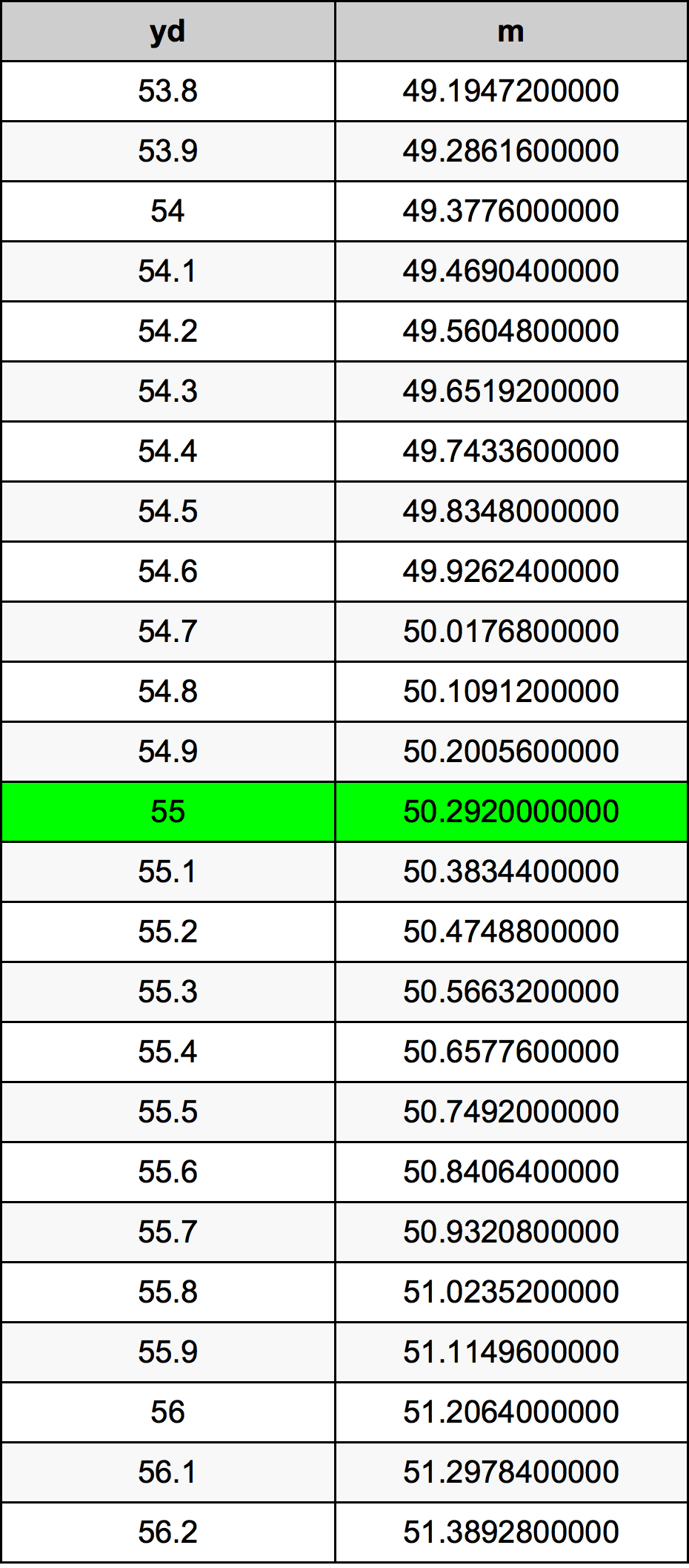Yards To Meters

# 55 yd to m55 Yards to Meters

yd
=
m

## How to convert 55 yards to meters?

 55 yd * 0.9144 m = 50.292 m 1 yd
A common question is How many yard in 55 meter? And the answer is 60.1487314086 yd in 55 m. Likewise the question how many meter in 55 yard has the answer of 50.292 m in 55 yd.

## How much are 55 yards in meters?

55 yards equal 50.292 meters (55yd = 50.292m). Converting 55 yd to m is easy. Simply use our calculator above, or apply the formula to change the length 55 yd to m.

## Convert 55 yd to common lengths

UnitLengths
Nanometer50292000000.0 nm
Micrometer50292000.0 µm
Millimeter50292.0 mm
Centimeter5029.2 cm
Inch1980.0 in
Foot165.0 ft
Yard55.0 yd
Meter50.292 m
Kilometer0.050292 km
Mile0.03125 mi
Nautical mile0.0271555076 nmi

## What is 55 yards in m?

To convert 55 yd to m multiply the length in yards by 0.9144. The 55 yd in m formula is [m] = 55 * 0.9144. Thus, for 55 yards in meter we get 50.292 m.

## 55 Yard Conversion Table## Alternative spelling

55 Yards to Meter, 55 Yards in Meter, 55 Yard to Meters, 55 Yard in Meters, 55 yd to Meters, 55 yd in Meters, 55 yd to m, 55 yd in m, 55 Yards to m, 55 Yards in m, 55 Yard to Meter, 55 Yard in Meter, 55 yd to Meter, 55 yd in Meter